# Playing Around with Elastic Collisions

This post is part of a series called You Do The Math.
Creating Generative Art with HYPE
Euclidean Vectors in Flash

In this tutorial we will create a game where the objective is to prevent other objects from colliding with your cursor. We won't use Flash's built-in hitTestObject() methods; instead we will write our own collision detection routines.

Republished Tutorial

Every few weeks, we revisit some of our reader's favorite posts from throughout the history of the site. This tutorial was first published in February of 2011.

## Final Result Preview

Let's take a look at the final result we will be working towards:

## Step 1: Start Off

Create a new Flash file (ActionScript 3.0)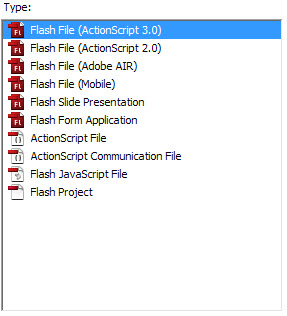Set the stage dimensions to 500x500px and FPS to 32.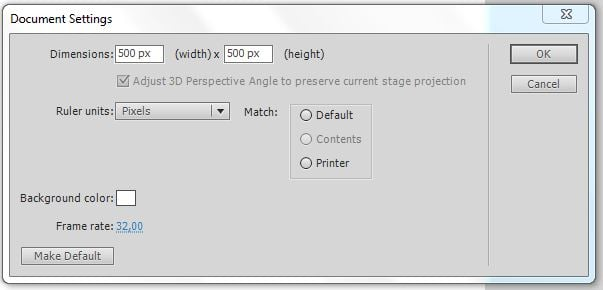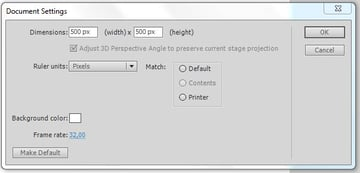## Step 2: The Ball Class

This class will contain all data related to one ball. A ball has a _mass, a _radius, an _xSpeed and a _ySpeed. So we will make a property for each. In the constructor we pass the mass, the angle and the speed of the ball. Because the class will be linked to a display object we can retrieve the the radius of our ball by dividing the width of the display object by 2. The _xSpeed and _ySpeed can be calculated by using simple sine and cosine functions.

For more information on these trigonometric Math.sin() and Math.cos() functions, see this Quick Tip.

## Step 3: Providing Getters and Setters

In our Ball class we provide getters and setters for our properties.

## Step 4: Update Function

This function updates the x and y properties of our ball according to the _xSpeed and _ySpeed. We'll implement this function in our Ball class.

## Step 5: The Completed Class

We'll finish up our Ball class in this step.

## Step 6: Display Objects for our Ball Class

In the source-files I included a start FLA which contains all the library items you need. You can draw them yourself if you want, of course. Make sure your FLA has the following display objects: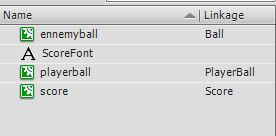(Ed. note: that's a typo: "ennemyball" should say "enemyball".)

## Step 7: Linking our Library Objects

The Ball class we just created has to be linked to the enemyball Sprite in the library.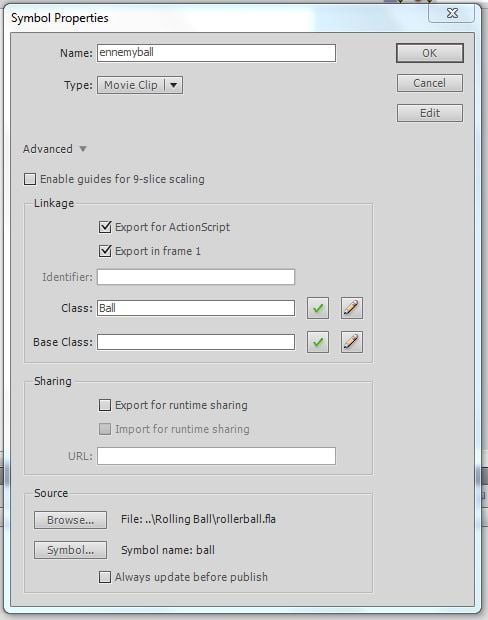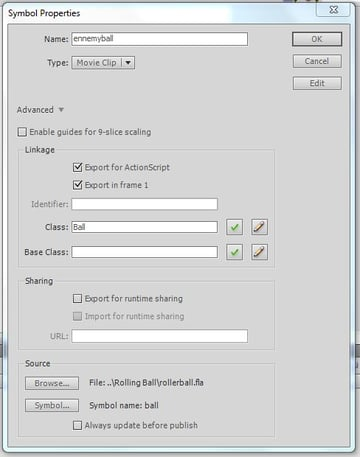The playerball Sprite must have Ball as base class and PlayerBall as class.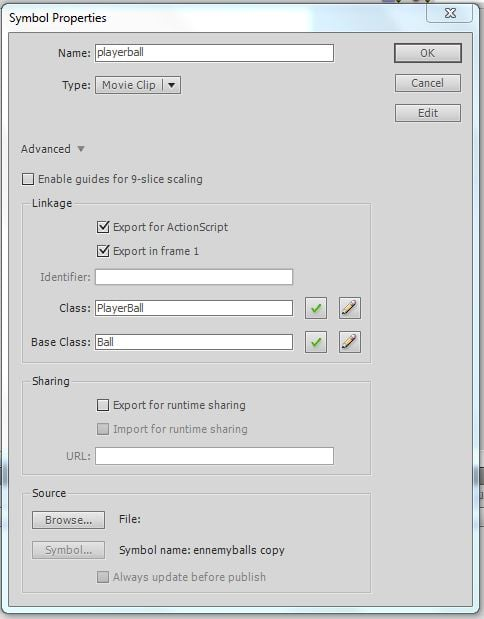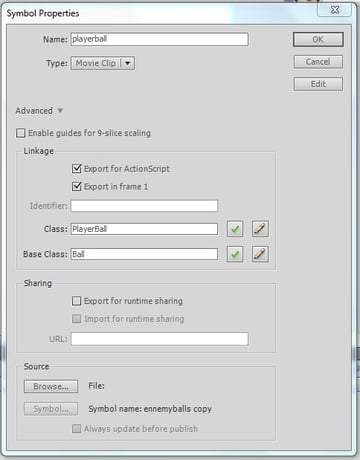The score movie clip must have a Score class.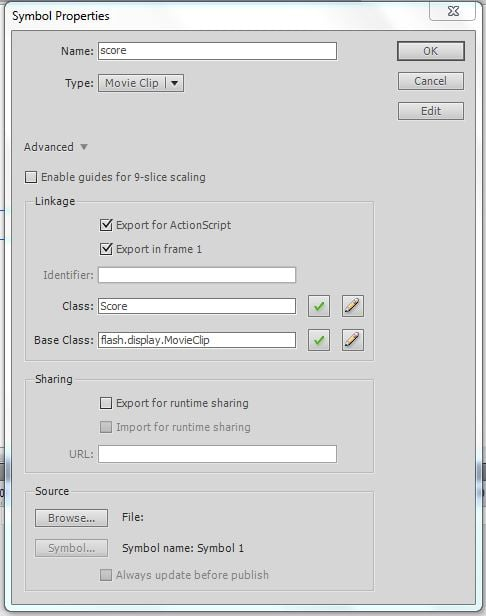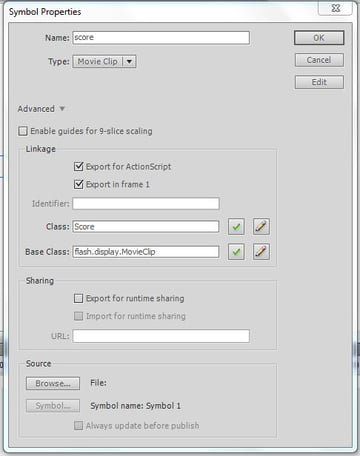## Step 8: The Application Class (Document Class)

The Application class will contain all the game logic. We import all the classes we need. As you can see we'll be using TweenMax.

Next we define our field variables. The first field variable is the ballPlayer.

Because the base class of our playerball Sprite is Ball we can store this Class in the ballPlayer variable. This makes it easier later on to check for collisions between the ballPlayer and the enemy balls.

The second field variable is an array which will contain all our enemy balls. The third variable is the timer that will be used to perform the main game loop. The fourth and last field is an instance of our Score library object that will be used to display the elapsed game time. In the constructor we call the init() function which I'll explain in the next step.

Don't forget to link the document class!.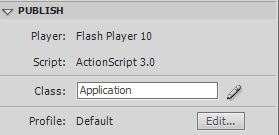## Step 9: The init() Function

Take a look at this code:

In the first four lines we initialize our field variables.

Next we make sure our stage is aligned to the top left corner and does not scale.

We hide the mouse cursor. Our cursor will be replaced with the playerball Sprite. Next we call the setBackground function(explained in the next step).

We center our score on the screen and add it to the display list. To update the position of ballPlayer we attach a MouseEvent.MOUSE_MOVE event to the stage.

The updatePlayerBall function (explained in step 11) will handle this MouseEvent. Next we add the ballPlayer to the display list.

The timer will be used to display the game time. We attach a TimerEvent.TIMER listener to our timer, which will trigger the updateTime() function (explained in Step 12) every 10 milliseconds.

Finally, we add a MouseEvent.CLICK to our stage. The startGame function (explained in step 13) will then start our game.

## Step 10: setBackground() Function

This function adds a radial gradient background to the display list. To draw a gradient on a Sprite you must define the type of gradient, the colors you want to use, alpha values of the colors, the ratios (these define the distribution of the colors)and the spread method.

## Step 11: updatePlayerBall() Function

This function updates the position of ballPlayer according to the position of your mouse.

## Step 12: updateTime() Function

We calculate the time in seconds and put it inside the textbox of our score Sprite. Every 5000ms (five seconds) we add a new ball to the game.

## Step 13: startGame() Function

The game is started by clicking the stage. First we remove the listener for the stage click, so that we can't start the game serveral times. We add three balls to the game by calling the addBall() function (explained in the next step) three times. We start our timer which will update our game time.

Finally we add an ENTER_FRAME event to our stage. The gameLoop() function (explained in Step 15) will update the position of our enemy balls.

First we make a new instance of our Ball class. We position the ball randomly on the stage with an alpha of 0 and add it to the display list.

Next we tween the alpha back to 1. (I use TweenMax, it's included in the source files. You can also use the built-in Flash tween engine.) The second tween isn't really a tween. It just waits a second and the onComplete function pushes the ball into our eballs array. This way the gameLoop() function (explained in the next step) can handle the rest.

## Step 15: gameLoop() Function

Every frame will go through this function.

We start by iterating through all of our enemy balls.

The second for-loop checks for collisions between the enemy balls. The loop starts at 'i + 1'. This way we don't double check the collisions.

Next we check if the ballPlayer hits the enemy ball. If so, the game is finished. Then we update the position of our enemy ball.

We make sure the balls stay in the game screen by calling the function checkBounds() (explained later on).

## Step 16: collision() Function

This function checks whether any given pair of balls are colliding.

First we calculate the x distance and the y distance between the two balls. Using Pythagoras's Theorem (see the following diagram) we calculate the absolute distance between them. If the distance is less or equal to the sum of the the radii of the balls we have a collision.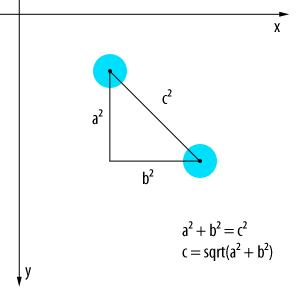## Step 17: doCollision() Function

This function will calculate the new x- and y-speeds of the balls according to the speed and angle of the collision. Warning: math ;)

First we calculate the horizontal distance between the two balls and then the vertical distance between the balls. With these distances (and a little more trigonometry) we can calculate the angle between the balls (see diagram).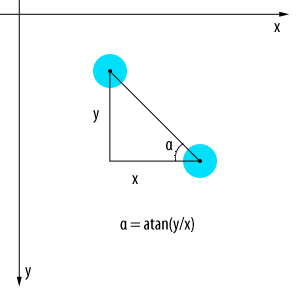Next we calculate what I call the magnitude of each ball. (We have an xspeed vector and a yspeed vector; the magnitude is the vector sum of those.) Then we calculate the angle of each ball (similar to the previous angle calculation).

Next we rotate the new x- and y-speeds of each ball. What we are actually doing is rotating the coordinate system. By rotating our axes we have a 1D collision. (See the following diagram).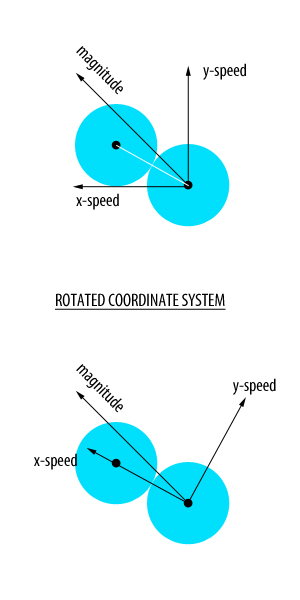Newton says that the total amount of kinetic energy in a closed system is constant. Now we use these formulae:

• v1 = (u1*(m1-m2) + 2*m2*u2)/(m1+m2)
• v2 = (u2*(m2-m1) + 2*m1*u1)/(m1+m2)

where:

v1 = final xSpeedBall 1

v2 = final xSpeedBall 2

m1 = mass ball 1

m2 = mass ball 2

u1 = initial speed ball 1

u2 = initial speed ball 2

The y-speeds don't change as it is a 1D collision.

With these formulae we can calculate the xSpeed and ySpeed of each ball.

Now whe have the new x- and y-speeds in our rotated coordinate system. The last step is to convert everything back to a normal coordinate system. We use Math.PI/2 because the angle between xSpeed and ySpeed must always be 90 degrees (pi/2 radians).

## Step 18: endOfGame() Function

This is run when the game ends.

First off all we stop the timer. We show the mouse again. Next we remove both the MOUSE_MOVE and ENTER_FRAME event listeners. Finally we make all the balls on the stage invisible.

## Step 19: checkBounds() Function

This function makes sure the balls stay inside the game screen. So if the ball hits the upper or lower side, we reverse the ySpeed. if the ball hits the left or the right side of the screen we reverse the xSpeed. It uses similar logic to the ball collision detection function to check whether the edges of the ball hits an edge of the screen.

## Step 20: The Complete Application Class

We have completed our Application class. We now have a working game!!!

## Conclusion

That's it for this tutorial. Of course you could add the possibility to restart the game, but that shouldn't be too hard. This basic example of elastic collisions can be used for bigger games like a billiards game or similar.

I hope you liked this tutorial, thanks for reading!